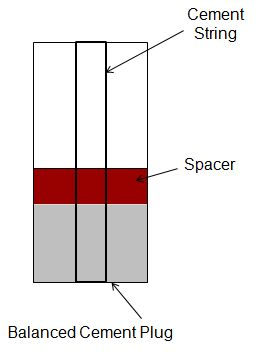## Balanced Cement Plug Calculation

You already learn about balance cement plug and I would like to show how to calculate balance cement plug.

Let’s start with the concept so you can imagine how the calculation should be.

1. Determine volume of cement that you need.

2. Determine height of cement and spacer when pipe in hole.

3. Determine displace volume to balance the hydrostatic both sides. When the displacement is completed, you should have equal height of cement/spacer/mud. You can see the figure below for more understanding.4. When you pull cement stinger, you will have the balance set properly like this.## Balanced Cement Plug

Balanced cement plug is the method to set cement plug by applying concept of balanced hydrostatic. Generally speaking, when we say “balance”, it means that hydrostatic pressure at the end of the drilling string is the same as hydrostatic pressure in the annulus (see the figure below).(Balance hydrostatic pressure both side)